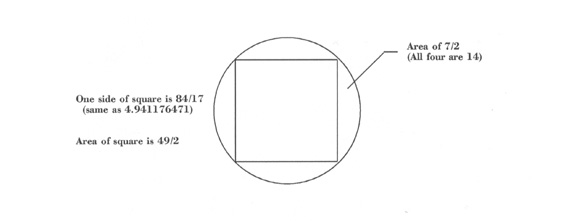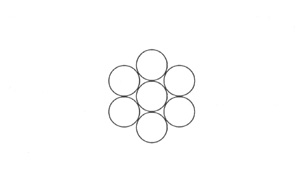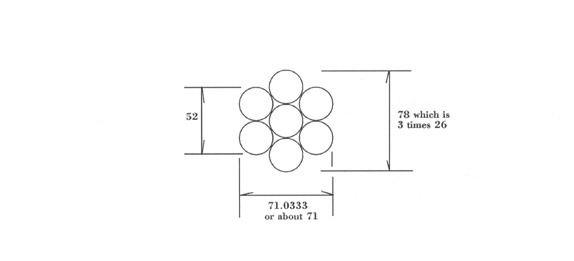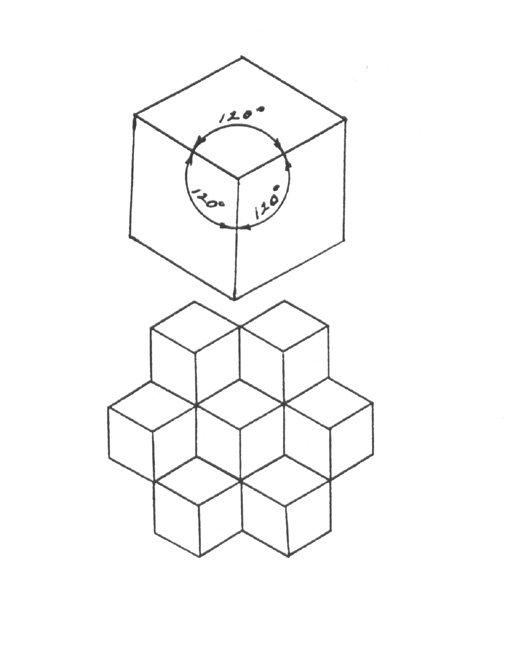### ASTROLOGY in the BIBLE Six

We can speculate on why the ancient Hebrew scholars chose to place coded messsages in what Christians call the Old Testament.   The presently conceivable possibilities are:

1.   A document was needed for initiates to decipher to attain their degrees.

2.   A time capsule was needed to show future generations how much was known in ancient times.

3.   A teaching device was needed for use by future generations who had lost the old knowledge.

4.   Some combination of the foregoing.

In any case, parts of the Pentateuch or Torah definitely resemble the message we sent into outer space several years ago in which universal mathematical knowns could teach aliens certain concepts about us.   This implies that we should be able to learn certain concepts about the ancient Hebrews from deciphering their writings.   And what have we learned so far from the few things we have viewed and analyzed from Genesis?   First, the following seem to be equivalencies

22 = the circumference of a circle

7 = the diameter of a circle

7/2 = the radius of a circle

49/4 = the radius of a circle squared

22/7 = pi

49/4 times 22/7 = the area of a circle

Remember that the river to south of Eden, called Gihon, sums to 77 which is the product of 11 and 7.   Eleven reduces to 2 and 7 is reduced as far as it will go.   Here we have another hint at the area of a circle.

If we have a circle that is circumscribed about a square:1.   The diagonal of the square is the diameter of the circle, which is 7.
2.   Half of the diagonal of the square is 7/2 which is the radius of the circle.
3.   The two diagonals of the square divide it into four equal parts.
4.   The area of two of these parts equals half the area of the square.   This area is equal to (7/2)2.
5.   Twice this area is the total area of the square which is 2(7/2)2 or 49/2.
6.   The total area of the circle is (49/4)(22/7) which is 77/2.
7.   The difference between the two areas is (77/2) - (49/2) which is 28/2 or 14.
8.   The area between 1/4 of the circle and 1/4 of the square is 7/2.
9.   Since the area of the square is 49/2, one side of the square is square root of 49/2 which is 7/(2)1/2 or 7 divided by the square root of 2.   This comes to 4.94974.   When this is expressed in fractional form it comes to about 84/17 which has a decimal value of 4.94118. In both cases, this is almost 5.

Do these numbers look familiar?   Eighty-four is the product of 7 and 12 which are the reductions of the land and river east of Eden.   Seventeen is one of the key numbers west of Eden (680 is the number of the western river, which is the product of 40 and 17).   And, interestingly enough, one side of our circumscribed square is almost exactly equal to 5, which makes the entire perimeter equal to about 20 (19.7647 to be precise).   By way of review, the following is the area near Eden:

NORTH
River is Pishon = 446 reduces to 14 and then 5
Land is Havilah = 64 which is 8x8, 8 squared, or
2 to the 6th power, reduces to 10 and then to 1.

WEST                                                             EAST
River is Euphrates = 680 reduces to 14 and then 5           River is Hiddekel = 142 reduces to 7
No Land                                         Land is Ashur = 507 reduces to 12 and then 3

SOUTH

River is Gihon = 77 reduces to 14 and then 5
Land is Cush = 326 reduces to 11 and then 2

In the center of this area is Eden which is equal to 124 (reduces to 7) which is a garden equal to 53 (reduces to 8).   One, two, four, and eight are the first part of a doubling series.   Sixty-four, the number of the land of Eden, hints at squares (64 equals 8 squared).   What could be important about a square?

Let us look at the east, the starting point for man, once more.   In the east is a land with a numerical value of 507 and a river with a numerical value of 142.   The difference between these numbers is 365 (507 - 142 = 365) which is the number of days in a year.   Could the square represent the four seasons of a year.   Squares also stand for four.   There are four years between leap years in which an extra day must be added to make up for the quarter of a day gain that occurs each year.   Actually a year is 365.2422 days long. Let us look farther.

The sum of the numbers of all the rivers is 1,345 (446+77+142+680=1,345).   The sum of the numbers of all the lands is 897 (64+326+507=897).   If we add the two sums we have 2,242 (1,345+897=2,242).   If we divide 2,242 by 365, the answer is 6 with a remainder of 52, the number of weeks in a year.   So 2,242 is six years expressed in days plus one more year expressed in weeks.   Six plus one is a seven year cycle (see article number four of this series).

If we subtract 897 from 1,345, the answer is 448, which is exactly equal to 7 times 64.   If 64 represents the idea of a square, we have seven squares indicated.   So perhaps seven years, each a square of four seasons, is the meaning.   If, on the other hand, 64 represents a cycle of four years, we have seven cycles of four years for a total of 28 years (about one orbit of Saturn).   Note also that the sum of the second reduction of the lands (6) and the rivers (22) is 28 which is 7 times 4 and the extension of 7.

Why a square?   There are four seasons in each year.   Each is 13 weeks long.   Seven (the number of days in a week) times 13 equals 91, and 91 is also the extension of 13 (see article four again on the Hebrew word meaning "breathed").   From this it seems that the circumscribed square refers to a year of four seasons.

However, observe what happens when we add the time line across Eden from east to west.   The sum of the river and land of the east plus Eden plus the reduction of the garden plus the sum of the river of the west (there is no western land) is 1,461 (142+507+124+8+680=1,461).   This number, 1,461, is the exact number of days in one four year cycle (four times 365 plus one leap year extra day).   So maybe the four means both seasons and four year cycle.

Now let us go back to the number seven.   Sixty-four is both a hint at seven because it is the seventh in the doubling series (see article five) and a hint at six because it comes from six doublings (two to the sixth power).   Seven has many significant correspondences.   It is the number of visible astrological planets, the number of days in one cycle (six days of creation plus one of rest), the number notes in our diatonic musical scale (which dates back to old Sumer), the number of spatial directions plus the center (used by the Hebrews to describe the universe - north, south, east, west, up, down, and the center), the number of years between major disruptions in one's life (see article four of this series), etc.   There is one meaning for seven which not been mentioned yet in this series.

Remember that the reference to seven of the sum 2,242 was by 365 times 6 plus 52?   The 52 weeks is a different way to refer to a year.   The six days of creation plus the seventh of rest is similar because the day of rest is different from the other six days.   When we speak of six directions plus the center, the center is different.

Seven is also the key to most biological cell arrangements and to structures such as the honeycomb.   Biological cells on a plane surface and the honeycomb of the bee are based upon six circles around a seventh (the different circle at the center).Is this figure also being described to us in Genesis?   The long distance across such a figure is three diameters.   These can be expressed as diameters of 7 or 26.   The number 326 of the southern land could mean 3 twenty-sixes.   The seven circle figure can be measured thusly:In article five, you can see where 71 is a factor of the eastern river.

If we were to use 7 as the diameter in lieu of 26, then we would be forced to use 19.1244 for 71.0333.   This would not be as wise because 19.1244 is not as close to a whole number.

The number 8 is found several times in the lands and rivers (see article five).   If we draw another circle around the seven circles, we will have eight circles in all.   There would be the large outer circle (macrocosm - the Eternal), the inner circle (mankind), and the six circles between (very ancient zodiac of only six signs).

The seven (or eight) circle diagram was one of the old ways to draw the tree of life, and the key to our base ten number system (see the book, THE TAROT by Paul Foster Case).   As a square inside the circle has a side of about 5, it is possible that either one season or one year (of the four year cycle) could be referred to as 5.   Then 20 could refer to either one year or four.

But let us look at the number 64 another way.   It is also representative of a cube because it is four cubed (4x4x4=64).   Seven times 64 is, possibly, a reference to seven cubes.   If we also consider 120 to be a number of degrees (446 - 326 = 120), we might find this number very significant.

Seven cubes hint at the beginning of a crystalline formation, the symbol of the earth plane (manifestation), the point of each cube being toward the viewer causing 120 degrees to appear between each line radiating from the point.Enough of number equivalencies and diagrams for now.   And what philosophical messages have been given to us so far in this little study?   Generally, we have been given some of the basics for a mathematical language and have been told the following.

Mankind moves along a time line, clearly described by an east/west diameter, and along a desire path not so clearly described but generally moving from south to north.   Terror moves him away from the south while the promise of wealth invites him to the north.   So his overall direction is from southeast to northwest.

Humanity has the astrological mechanism to prod him along as it interacts with his memory patterns.   This mechanism is the key to man's growth and development.

There is a seven year cycle and each seventh year is different from the other six years.   Seven is a key to our growth, both physically and otherwise.   There is an annual seasonal cycle and a four year cycle.   There is a 28 year cycle composed of four seven year cycles or seven four year cycles.

And, of course, there are all the more complex messages mentioned in article four.

The wonder of it all is the extreme volume of information that can be expressed in only a few numbers and their relationships to one another...